# Dividing a sphere into twelve "identical" shapes.

## Recommended Posts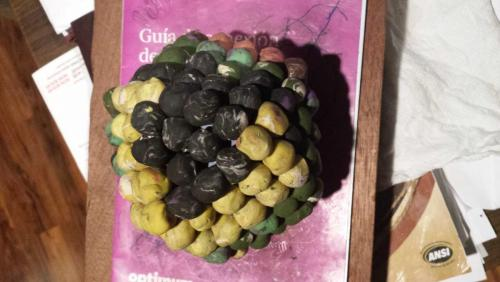Built out to 4th level

first level 12, one sphere per segment total 13

second level 42, 3.5per segment total 55

third level 92, 7.67per segment total 147

fourth level 162, 13.5per segment total 309

Edited by tar

• Replies 184
• Created

#### Popular Days

total sphere population per segment counting all levels at each level (appox.)

1st 1.08

2nd 4.583

3rd 12.25

4th 25.75

Edited by tar
##### Share on other sites

The figure built out in half sized little balls, to the 4th level, yields the cube octahedron. This could be divided into the 12 segments, using each of the corners of the cube octahedron as the center of each segment. Best way to translate this to the globe setup is put a corner on the equator, go diagonally across a square side, and put that corner on the equator as well. Each diamond shaped segment would be composed of a third of one triangular side, a quarter of the square side CCW around the corner, a third of the next CCW triangle and a quarter of the next CCW square side.

In the picture here, I show the 9 hexagon layers, and the 9 square layers, evident when you build the figure out, to the 4th shell.

Where only 5 square layers are shown, 4 repeat in flipped orientation in the stack of 9.

Same for the 9 hexagonal layers, 4 repeat in a flipped fashion.

This stack of 9 square layers happens three different times, as you could set the figure on six different sides. The stack of 9 hex planes happens 4 different times, as you could set the figure on 8 different triangular sides.

Regards, TAR

or 63 uniquely membered plane sections 3x9 square layers and 4x9 hexagonal layers 27 and 36

Edited by tar
##### Share on other sites

• 3 weeks later...

Built out to the 5th level.

252 balls in the outside shell.

There are many 5 step routes, always going away from the center, to a ball on the outside shell, always traveling to a touching ball. This is demonstrable on any full hex plane, going through the center and on any full square plane going through the center. I believe it is also possible to find 5 step routes, always traveling outward to touching balls, but shifting between planes.

Regards, TAR

1,12,42,92,162,252

after 12 the progression is 30,50,70,90

##### Share on other sites

Just lost an explanatory post. Will fill in the details later, but here is the figure built with 12 great circles, really 6 since the same circle is both the great circle that goes from the red toothpick through the yellow as the one that goes from the yellow toothpick through the red.

Purpose is to begin to relate the TAR coordinates to other coordinate systems. The three points on the sphere are marked by toothpicks. Four colors, 8 points, consistent with the corners of a cube.

The red cube has a dot on the facing edge, which corresponds to the center of segment number 1.

The cube is cut in half horizontally by the xz plane. The yz plane cuts the viewer in half vertically cutting the cube lead edge to far edge, and the xy plane cuts the cube in half corner to corner.

Regards, TAR

Edited by tar
##### Share on other sites

Each North toothpick can be thought of as North axis point of its six segment color wheel.

The degrees would go around like lines between the segments of a tangerine. Although this particular tangerine would have virtual segments for each of the four colors. The degree lines would run from the north end of that color toothpick, to the south end. The important part of the lines would be those segments of the lines within the six segments making up the "wheel" of that color.

For instance, the great circle going through red and green is the 330 degree marker on the border between the number 6 and number 1 red segment, and also, coming up the other side, the 150 degree line between the number 3 red segment and the number 4 red segment.

The degrees proceed around the North red toothpick CCW with 0/360 being in the center of segment 1.

The degrees proceed around the North yellow toothpick CW with 0/360 being in the center of segment 1.

Around the north blue CCW with 0/360 of the blue wheel being in segment 11.

And CW around the green north, with 0/360 for the green wheel, also being through the center of segment 11.

Of course the south ends of the toothpicks would appear reversed with the red degrees proceeding CW, the yellow CCW, the blue CW and the green CCW.

Regards, TAR

Edited by tar
##### Share on other sites

• 4 weeks later...

Each color "wheel" divided into 6 60 degree segments. The center of the number 1 segment holds R0/360 and Y0/360 with the degrees proceeding up and to the right for red and up and to the left for yellow. The number 11 segment holds the 0/360 point in its center for the blue and green "wheel", with the degrees proceeding up and to the right for blue and up and to the left for green.

Regards, TAR

pictures came in rotated

put the balloon tail at the south pole for bearings in terms of what is up and right and left for segment 1 and 11

Edited by tar
##### Share on other sites

• 1 month later...

Interesting to me, was a recent finding, that making same size circles centered on the three points and 4 points of the 12 diamond figure, yielded 14 circles...and that dividing each diamond in half both ways, or into 4 sections, and then drawing same size circles yielded 50 circles...and splitting each segment 3 ways and doing the same yielded 110 circles.

12 segments yielded 14. Splitting each in half both ways gives 12 times 4 or 48 segments, but centering the circles on the intersections yielded 50, or two more, same as the difference between 12 and 14. Then splitting each section 3 ways yielded 9 times 12 or 108, yet the count of the circles centered on the intersections was 110, again, 2 more.

I speculate that should I divide each section 4 ways to get 16 times 12 or 192, if I drew circles on the intersections, I would guess the count would be 194.

Just interesting, that a sphere is thusly arranged

I have yet to prove to myself that the circles are all the same size, but for instance on the 14 count sphere, each circle has a radius of one half a diamond side, which makes them all the same size. The higher count circle's consistent radii would depend on the division lines being the same length as the sides. I have yet to prove that is the case, but the division lines are segments of great circles, even distance from each side of the diamond...I have not proved yet that this requires that all such division lines are equal length.

Regards, TAR

For clarity, the balloons have colored lines that are the division lines I am talking about, as well as a full circumference line of the same color which is drawn to show such a circumference completes the color wheel in each case, but the resulting line at right angle to the division lines, is NOT the same length as a side of a diamond or as one of the division lines I am postulating may well be the same length as the side of the diamonds.

##### Share on other sites

• 1 month later...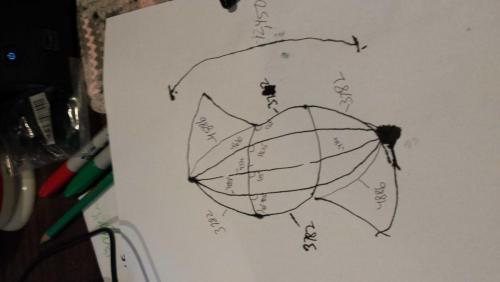Just lost a long descriptive post, so I am posting these pictures and then I will edit in the description so as not to lose my work again.

rats lost it again,

Anyway it looks like the division lines are the same length as the sides of the diamonds.

Regards, TAR

One half a great circle would consist of the side of two diamonds and one length of a diamond's short diagonal.

Using the Earth diameter of 7926 we would have diamond sides of the length 3782. Or .955 of a radius. The diamond short diagonal would be 4886 and adding a side to a diagonal and dividing by two you would get 4334. So happens 4334 plus 4334 plus 3782 is 12450 so it looks like the center division line could easily be the 3782. If the edges are 3782 and the center is 3782 it looks like the 1/4 and 3/4 marks would also be 3782. The length between the mark and the axle would be 4058 and 4610 for the 1/4 and 3/4 mark on the top edge of the diamond and vice a versa on the bottom.

The angles between the masking tape and the great circle division lines seems to be proportional as well. Going 90, 97.5, 105, 112.5 120 from 4point, 1/4, 1/2, 3/4 the way and 3point.

Edited by tar
##### Share on other sites

notice that as the angle smoothly changes from 90 degrees to 120 degrees as you go across a side from 90 degrees to 120 degrees, the distance between the mark and the axle point of wheel also changes smoothly from 3782 to 4886. Halfway between you would expect the average of 3782 and 4886 which is 4334. The quarter mark would be the average of 4334 and 3782 which is 4058 and the average of 4334 and 4886 is 4610. Checking back 3782 plus 3782 plus 4886 is 12450. 4058 plus 3782 plus 4610 is 12450. 4610 plus 3782 plus 4058 is 12,450 and finally the other edge 4886 plus 3782 plus 3782 is 12450.

##### Share on other sites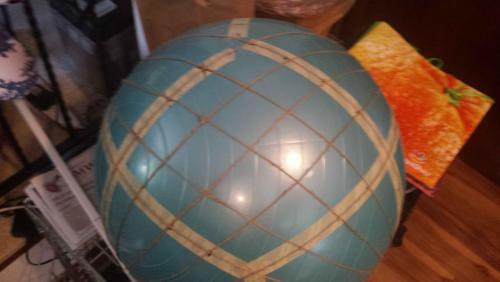Thread, the division lines seem to be equal length but the divisions are not regular. Of the 16 you get when dividing the section into 15 degree smaller diamonds there seems to be 6 versions. The two touching the 120 angle are more shield shape than diamond shape. The two at the 90 degree corners are mirror images of each other, the four that touch these two are in turn mirror images of each other, and the four touching the shield sections are mirror images of each other (reflected off the short and long diagonals.)Left are the 4 in the middle, the two touching vertically seem to be similar and the two touching horizontally seem to be similar.

My exercise ball was done by eye and is rather crude, so I have not determined what the angles of these various diamonds are...nor if each diamond has a similar surface area, which would be the crucial characteristic if this system were to be used as a regular way to divide up the sphere.

Regards, TAR

Edited by tar
##### Share on other sites

Actually the angles come out quite regular, and one could figure any divisions just starting with the 120 and 90 and assuming a smooth proportional change in the angles.

Each intersection has to add up to 360 and since two lines are crossing you only need one angle to figure the other three in the intersection.

Regards, TAR

Still, here with 15 degree divisions you have six differently shaped areas and therefore differently shaped volumes. Although I am still working on whether the surface area and volumes are equal from one differently shaped diamond to the next.

##### Share on other sites

Maybe I'm a layman, but wouldn't a sphere cut into 12 wedges been easier?

##### Share on other sites

Raider5678,

Easier, yes.

But not as many symmetries and dual figures, and it doesn't build out to fill space...well I guess it would, but not as interestingly.

Regards TAR, a layman as well

Edited by tar
##### Share on other sites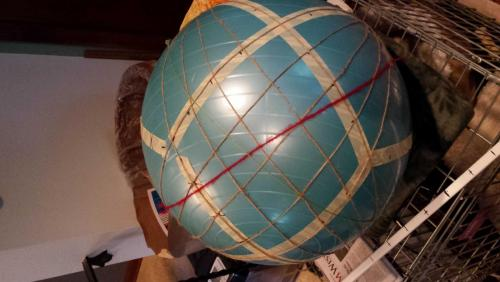Although the area of the segments may not be uniform, the scheme still allows for identifying every point on a sphere with two coordinates. Pictured very faintly here is a green yarn and a more noticeable red yarn piecee The segment shown is segment number two with the North pole laying to your left. The red thread is the portion of the

circumference of the red wheel from 30 degrees R to 90 degrees R from bottom left to upper right. The faint green line indicates the portion of the green circumference between 30 degrees G to 90 degrees G going from upper right to lower left.

Therefor any number of degrees G between 30 and 90 along with any number of degrees R between 30 and 90 will designate one and only one point within this shown diamond.

Each degree or portion thereof would designate one point on the designated circumference and would be associated with a great circle going through that point.

Each degree or portion thereof on the other color circumference would be associated with a great circle going through that point. These great circles are shown by the threads, which are positioned every 15 degrees within the 60 degree portion of the red wheel and the 60 degree portion of the green wheel which define segment 2.

Thus where two threads cross is a single named point on the surface of the sphere. The threads are material representations of hypothetical great circles through the axis of a colored wheel crossing its circumference at two points, once creating a division line in segment 2 in this picture and once in segment 4 on the other side of the sphere.

Regards, TAR

sorry my picture rotated, the north pole is at the bottom, not to the left.

Turn the picture 90 degrees CW for my description to jive.

actually even when rotated my description is wrong, the red runs from lower left to upper right and the green from lower right to upper left

In the scheme the number one segment is like you are looking at the equator and there is a segment in front of you with the upper case R numbers starting in the center of the segment in front of you and proceeding p an to the right. The Y numbers start in the same spot and go up and to the left. If you follow the equator to the right you arrive at the 11 segment where the Blue wheel starts up and to the right and the Green wheel starts up and to the left.

Edited by tar
##### Share on other sites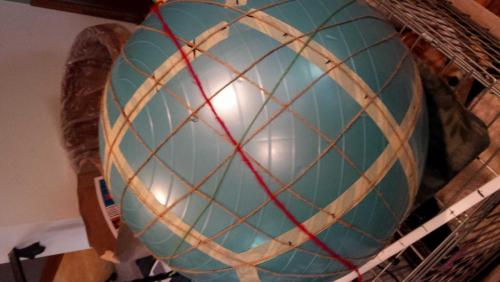In measuring the angles of the crossing of the red and the green circumference lines, I noticed that I had just let the yarn sit where it fell and had not "adusted" it to meet any preconceived notions. However I noticed that the lines are suppose to go through the center of the diamond, as this is the definition of the circumference line that creates the wheel of 6 diamonds making up a color wheel.

Once adjusted, I noticed that the angles are 105 and 75 just like the division lines in the center, but switched to where the 75 angles are oriented 90 degrees differently. But both the division lines and the circumference lines meeting in the center of the diamond both measure 75 and 105 degrees and logically should be 75 and 105 degrees.

This may assist in determining if the division lines create equal area diamonds.

Regards, TAR

again, conceptually it is better to rotate this picture 90 degrees CW, putting the North pole on top, for consistency in visualizing this segment number 2 and its relationship to the rest of the sphere

##### Share on other sites

Interesting development.

I was thinking about the balloon with the equal size circles on the intersections of the division lines. Each segment contains portions of four circles and the area between four touching circles...

Lost a big section of my post here, but here is the main idea.

Add up the angles inside each of the divisions. In the case of the 15 degree divisions there are 16 in a diamond. In the case of 16 in a diamond each adds up to 363.75. That means, if we assume each division line is of equal length within the diamond, and the circles earlier drawn on the intersections are equal because their radii are equal, then each of the 16 divisions of the diamond is of the area 363.75/360th of one of these circles, plus the area between four touching circles.

Double checking, I am thinking about the "two extra" thing earlier run across. 15 degree divisions would make 16 per diamond times twelve or 192. 192 divisions and 194 circles which would mean there should be 194/12 circles within each diamond... which is 16.16666 circles per diamond. 16.16666 divided by 16 is 1.01041625. 1.0104165 times 360 is 363.74985, which without the abbreviated numbers would actually come out to exactly the 363.75 we have found is the case.

Regards, TAR
check with drawing posted in 162
every four number collection found inside a division adds up to 363.75

Edited by tar
##### Share on other sites

Here is first draft workup of the number of internal degrees that each division shield/diamond will add up to, depending on how many degrees per division, in terms of the color wheel.

For instance, with 20 degree divisions, one main 60 degree diamond would be divided up into 9 divisions, each 20 degrees by 20 degrees.

+

In that case there would be 9x12 or 108 divisions and 110 circles on the sphere. Each division would have the area of 110/108th of a circle, plus the area in between 4 touching circles. So the circle area in each division would be the total of the 4 pieces of circle found in the division. In the case of the 20degree divisions, which is 9 divisions per main 60 degree diamond, the total of the internal angles in each division will be 266.666(666repeating). For instance 110+73.333+80+103.333=366.666

Regards, TAR

##### Share on other sites

Way back in the thread I gave directions to draw the 12 diamonds out of 12 strokes...I think. But here are the directions again, possibly more complete, now knowing that the side of a diamond is .955 (approx.) of a radii of the sphere.

Draw or inscribe or use tape or string or whatever, but here is an easy way. Prepare 12 lengths of your media, .955 the diameter of the sphere you wish to demarcate. Mark a North pole, a South pole, and a prime meridian (a fixed point on the equator of the sphere, halfway between N and S) , with small removable or coverable marks. Make a plus sign with two of the diameters and place the center on the North pole, with one "arm" pointed directly toward the meridian mark and the South pole. This establishes the exact position of the other 5 plus signs you can make from the remaining 10 diameters. Place the second cross on the South pole, with one of the arms headed directly toward the meridian line (and the North pole.) Now think of the next cross as an X and put its center on the equator, but not on the meridian mark, but 45 degrees to the left or right, so that all four ends exactly touch the ends of two arms coming from the North and two arms coming from the South. Do the same to the right or left of the meridian mark with the 4th plus sign. The position of the 5th and sixth plus signs are obvious from here.

Regards, TAR

The meridian mark will be right in the center of diamond number 1 with diamond 2 and the red wheel going up and to the right. Up and to the left is diamond 7 and the second diamond in the yellow wheel. The diamond to the right, along the equator from the number one diamond is the number 11 diamond which is the origin and end point of the blue wheel, going up and to the right (to the number 3 diamond), and the green wheel, going up and to the left (to the number 2 diamond.)

Edited by tar
##### Share on other sites

• 3 weeks later...

The divisions which we now know (sort of proved, with the exception of the consistency of the area between four circles) to be the same area per division, regardless of the whole number division of a diamond length, can be labeled with the coordinates, of the one corner of the small (shield/diamond) area consistent with the direction from which the color wheel degrees are progressing. Shown here with the cyan dot in the base corner. The capital letters RYBG and the little letters rybg would be used to show when the arrow for a particular color is headed North or South. The numbers on this yellow balloon are all drawn to where their tops are facing North and their bottoms are facing South.

The divisions I found are not easy to follow in this fashion when there are an odd number of divisions but it works out nicely when there are even numbers.

So depending on what you are dividing up the sphere to do, you can divide it up in equal area diamond/shields, according to a particular even number division of a diamond length. Smaller still than one degree per side can be achieved by using the minute, second convention. It would be required to keep it at whole numbers though, as 60 is an even number and workable in this plan. Decimals would not work for going smaller.

Regards, TAR

A degree by a degree would be about 4,559 square miles if the scheme would be applied to the Earth. A minute by minute would be about 1.266 sq. mi. and a second by second would be about 9,806.48 sq. ft. or close to 99 ft. by 99 ft.

Edited by tar
##### Share on other sites

(just noticedthe smiley face on the medium size division paper and wanted to let you know it has nothing to do with the scheme. I was in the hospital getting a knee replaced and have to inject ​a blood thinner into my stomach in a little smiley face pattern two inches from my belly button, and that little drawing is a picture of where to place the injections.)

##### Share on other sites

• 4 weeks later...

Still trying to determine if the area between the circles is of equal area in each division. On the way I have applied the circles, or ovals to the triangle sphere I developed earlier, with the shield shapes that show themselves when you divide a tetrahedron into the twelve sections. So it is like the four sides of the tetrahedron unfolded and laid flat to give a two dimensional view of a sphere, looking at all sections at once.

This to show the "plus two" nature of the sphere and its divisions when you compare the number of circles on the intersections of the divisions with the number of divisions.

Here, the three points of the diamond make up one circle, the sides, since folded over have half circles on them, and the center half circle on each side is actually a full circle, since the center of each side represents a point of the tetrahedron where the circle is around a three point, like at the points of the large triangle.

Here you can see how the sphere comes around on itself and will have two more circles on the division intersections, then it does have divisions.

Regards, TAR

Edited by tar
##### Share on other sites

Sorry for not reading the entire thread... I have drifted in and out occasionally, but then I thought - hold on, I must be stupid or something.. why not just slice it in half. Slice those halves into 3 segments each. Then slice each in half again to get 12 exactly equal slices of the sphere - like orange segments. You could slice the last 6 segments in half either vertically or into 2 half segments each to get 2 different solutions.

What am I missing here?

PS - apologies for my ignorance here, but what am I missing?

Edited by DrP
##### Share on other sites

DrP,

There are symetries and dual figure relationships I am exploring that are very satisfying to me. More than orange slices. I have always struggled mightily with the sphere, and its geometry and am looking for a system that deals more in whole numbers and fractions, than approximations, to label the positions on the sphere, and the area and volume as well.

I could not divide the orange slice smaller and smaller in such a regular fashion as I can the twelve diamond sections.

Regards, TAR

##### Share on other sites

• 8 months later...

I have finally proven to myself that the divisions of the diamond, are of equal area, hence volume, hence can be divided into finer and finer measure, as desired, and my system can be used to denote, any particular area or any particular direction, given a sphere of defined radius, with a north pole and a point on the equator being the center of diamond #1. The four wheels, red, yellow, blue and green each 360 degrees, allow for the definition of the bottom corner of an area of whatever fineness you need.

Using the chart and diagram in post #162 and this calculator (named rechner, meaning one who calculates, on a german site) https://rechneronline.de/pi/spherical-triangle.php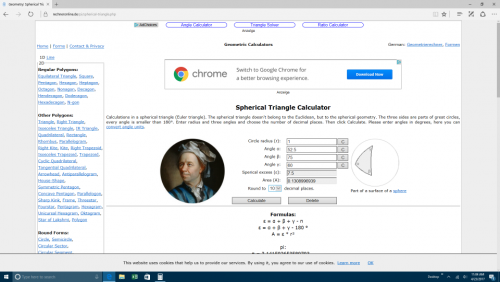you can find half the excess and area of the sections to be 1.875 and .0327249235 to 10 digits respectively

in the example of 16 sections per diamond (15 degree divisions) you multiply these numbers by 32 and then 12 and you get 720 and 4 times pi respectively

the eight sections on the diagonals can be figured directly by bisecting the non duplicated angles

the other 8 cannot be done in this fashion but can be figured by figuring a quadrant subtracting the two known areas, and dividing the remainder in half (bisecting the angles with a plane does not go through the center of the sphere with these eight)

it works with a diamond, and with a quartered diamond and with this example of the 1/16 of a diamond area

I postulate that any degree by degree division will work out to be of equal area, even the shield shaped sections up against the 120 angle corners of the diamond.

Regards, TAR

for instance the excess of a diamond degree would be 720 divided by the number of diamond degrees which is already established as 60x60x12 or 43,200. or an excess of 0.01666666666666666666666666667 per TAR diamond degree or an area of 2.908820866572159615394846141477e-4 given a sphere with the radius of 1

(thank you Leonhard Euler)

Edited by tar

## Create an account or sign in to comment

You need to be a member in order to leave a comment

## Create an account

Sign up for a new account in our community. It's easy!

Register a new account